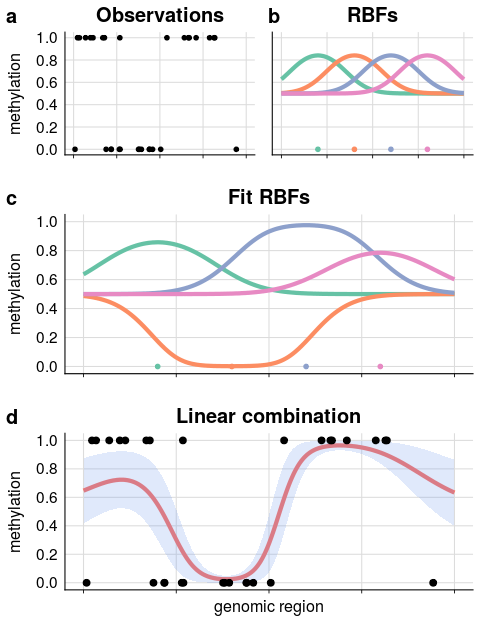# 1 Installation

## try http:// if https:// URLs are not supported
if (!requireNamespace("BiocManager", quietly=TRUE))
install.packages("BiocManager")
BiocManager::install("BPRMeth")

## Or download from Github repository
# install.packages("devtools")
devtools::install_github("andreaskapou/BPRMeth", build_vignettes = TRUE)

# 2 Introduction

DNA methylation is probably the best studied epigenomic mark, due to its well established heritability and widespread association with diseases. Yet its role in gene regulation, and the molecular mechanisms underpinning its association with diseases, are still imperfectly understood. While the methylation status of individual cytosines can sometimes be informative, several recent papers have shown that the functional role of DNA methylation is better captured by a quantitative analysis of the spatial variation of methylation across a genomic region.

The BPRMeth package is a probabilistic method to quantify explicit features of methylation profiles, in a way that would make it easier to formally use such profiles in downstream modelling efforts, such as predicting gene expression levels or clustering genomic regions according to their methylation profiles. The original implementation  has now been enhanced in two important ways: we introduced a fast, variational inference approach which enables the quantification of Bayesian posterior confidence measures on the model, and we adapted the method to use several observation models, making it suitable for a diverse range of platforms including single-cell analyses and methylation arrays. Technical details of the updated version are explained in .

In addition to being a flexible tool for methylation data, the proposed framework is in principle deployable to other measurements with a similar structure, and indeed the method was already used for single-cell chromatin accessibility data in .

# 3 Background

Mathematically, BPRMeth is based on a basis function generalised linear model. The basic idea is as follows: the methylation profile associated to a genomic region $$D$$ is defined as a (latent) function $$f\colon D\rightarrow (0,1)$$ which takes as input the genomic coordinate along the region and returns the propensity for that locus to be methylated. In order to enforce spatial smoothness, and to obtain a compact representation for this function in terms of interpretable features, we represent the profile function as a linear combination of basis functions

$f(x)=\Phi\left(\mathbf{w}^Th(x)\right)$

where $$h(x)$$ are the basis functions (Gaussian bells by default), and $$\Phi$$ is a probit transformation (Gaussian cumulative distribution function) needed in order to map the function output to the $$(0,1)$$ interval. The latent function is observed at specific loci through a noise model which encapsulates the experimental technology.Figure 1: Illustration of the process for infering methylation profiles using the BPRMeth package

The optimal weight parameters $$\mathbf{w}$$ can be recovered either by Bayesian inference or maximum likelihood estimation, providing a set of quantitative features which can be used in downstream models: in  these features were used in a machine learning predictor of gene expression, and to cluster genomic regions according to their methylation profiles. Modelling details and mathematical derivations for the different models can be found online: http://rpubs.com/cakapourani.

# 4 Analysis Pipeline

The workflow diagram and functionalities of the BPRMeth package for analysis of methylation profiles are shown in Figure 2.